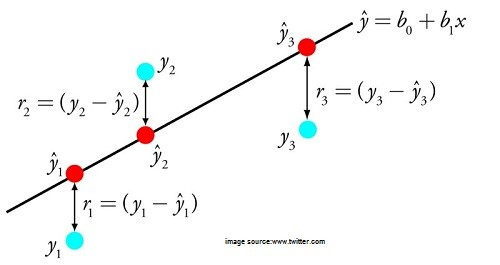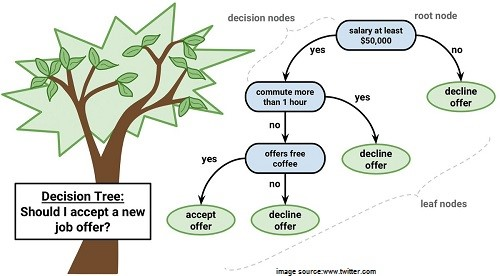# Top Artificial Intelligence Technologies

12714By John White Last Updated on Jun 15, 2021
•• Categories AI & Machine Learning

Linear Regression

Linear regression is used in mathematical statistics for more than 200 years as of now. The point of the algorithm is finding such values of coefficients (B) that provide the most impact on the precision of the function f we are trying to train.

The simplest example is y= B0 + B1 * x, where B0 + B1 is the function in questionBy adjusting the weight of these coefficients, the data scientists get varying outcomes of the training. The core requirements for succeeding with this algorithm is having clear data without much noise (low-value information) in it and removing the input variables with similar values (correlated input values). This allows using a linear regression algorithm for gradient descent optimization of statistical data in financial, banking, insurance, healthcare, marketing, and other industries.

Suggested Read: Artificial Intelligence trends for 2019

Logistic Regression

Another popular AI algorithm is Logistic regression, able to provide binary results. This means that the model can both predict the outcome and specify one of the two classes of the y value. The function is also based on changing the weights of the algorithms, but it differs due to the fact that the non-linear logic function is used to transform the outcome. This function can be represented as an S-shaped line separating the true values from false ones.

The success requirements are the same as for linear regression — removing the same value input samples and reducing the quantity of noise (low-value data). This is fairly an easy function that may be relatively fast and is great for executing the binary classification.

Linear Discriminant Analysis (LDA)

This is a branch of the logistic regression model that can be used when more than 2 classes can exist in the output. Statistical properties of the data, like the mean value for every class separately and the total variance summed up for all classes, are calculated in this model. The predictions allow to calculate the values for each class and determine the class with the most value. To be correct, this model requires the data to be distributed according to the Gaussian bell curve, so all the major outliers should be removed beforehand. This is a great and quite simple model for data classification and building the predictive models for it.

Decision TreesThis is one of the oldest, most used, simplest and most efficient ML models around. A classic binary tree with Yes or No choice at each split until the model touches the result node. This model is simple to learn, it doesn’t require data normalization and can help to solve multiple types of problems.

Naive Bayes

Naive Bayes algorithm is a simple, yet very strong model for solving a wide variety of complex problems. It can calculate 2 types of probabilities:

1. A chance of each class appearing
2. A conditional probability for a standalone class, given there is an additional x modifier.

The model is called naive as it operates on the assumption that all the input data values are unrelated to each other. While this cannot take place in the real world, this simple algorithm can be applied to a multitude of normalized data flows to predict results with a great degree of accuracy.

K-Nearest Neighbors

This is fairly an easy and powerful ML model, using the total training dataset as the depiction field. The predictions of the outcome value are calculated by checking the whole data set for K data nodes with similar values (so-called neighbors) and using the Euclidian number (which can be easily calculated based on the value differences) to determine the resulting value.

Such datasets can require lots of computing resources to store and process the data, suffer the accuracy loss when there are multiple attributes and have to be constantly curated. However, they work extremely fast, are very accurate and efficient at finding the needed values in large data sets.